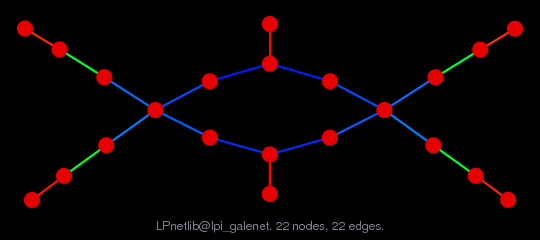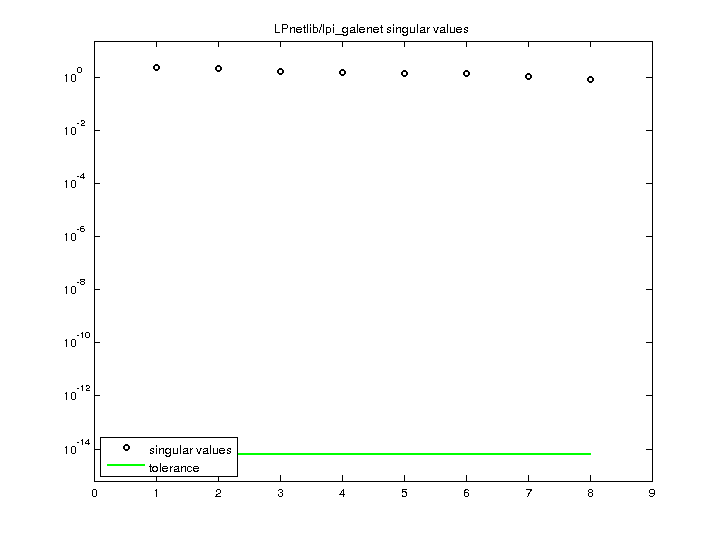Matrix: LPnetlib/lpi_galenet

Description: Netlib LP problem galenet: minimize c'*x, where Ax=b, lo<=x<=hi(bipartite graph drawing)• Matrix group: LPnetlib
• download as a MATLAB mat-file, file size: 1 KB. Use UFget(715) or UFget('LPnetlib/lpi_galenet') in MATLAB.

 Matrix properties number of rows 8 number of columns 14 nonzeros 22 structural full rank? yes structural rank 8 # of blocks from dmperm 1 # strongly connected comp. 1 explicit zero entries 0 nonzero pattern symmetry 0% numeric value symmetry 0% type integer structure rectangular Cholesky candidate? no positive definite? no

 author H. Greenberg editor J. Chinneck date kind linear programming problem 2D/3D problem? no

 Additional fields size and type b full 8-by-1 c full 14-by-1 lo full 14-by-1 hi full 14-by-1 z0 full 1-by-1

Notes:

```An infeasible Netlib LP problem, in lp/infeas.  For more information
send email to netlib@ornl.gov with the message:

send index from lp

The lp/infeas directory contains infeasible linear programming test problems
collected by John W. Chinneck, Carleton Univ, Ontario Canada.  The following
are relevant excerpts from lp/infeas/readme (by John W. Chinneck):

PROBLEM DESCRIPTION
-------------------

GALENET:  a very small network problem.  Contributor:  H.J.  Greenberg,

Name       Rows   Cols   Nonzeros Bounds      Notes
galenet       9      8       16   B

```

 Ordering statistics: result nnz(V) for QR, upper bound nnz(L) for LU, with COLAMD 31 nnz(R) for QR, upper bound nnz(U) for LU, with COLAMD 17

 SVD-based statistics: norm(A) 2.42798 min(svd(A)) 0.841189 cond(A) 2.88637 rank(A) 8 sprank(A)-rank(A) 0 null space dimension 0 full numerical rank? yes

 singular values (MAT file): click here SVD method used: s = svd (full (R)) ; where [~,R,E] = spqr (A') with droptol of zero status: ok